transreduction

Transitive reduction

Description

example

H = transreduction(G) returns the transitive reduction of graph G as a new graph, H. The nodes in H are the same as those in G, but H has different edges. H contains the fewest number of edges such that if there is a path from node i to node j in G, then there is also a path from node i to node j in H.

Examples

collapse all

Create and plot a complete graph of order four.

G = digraph([1 1 1 2 2 2 3 3 3 4 4 4],[2 3 4 1 3 4 1 2 4 1 2 3]);
plot(G)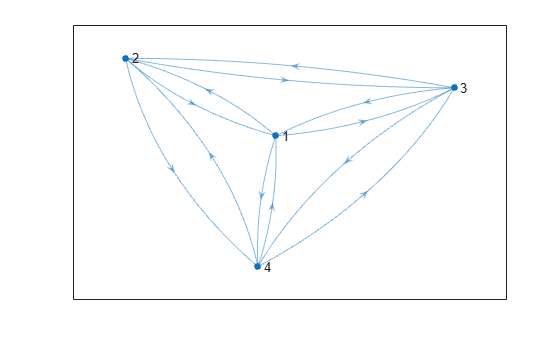Find the transitive reduction and plot the resulting graph. Since the reachability in a complete graph is extensive, there are theoretically several possible transitive reductions, as any cycle through the four nodes is a candidate.

H = transreduction(G);
plot(H)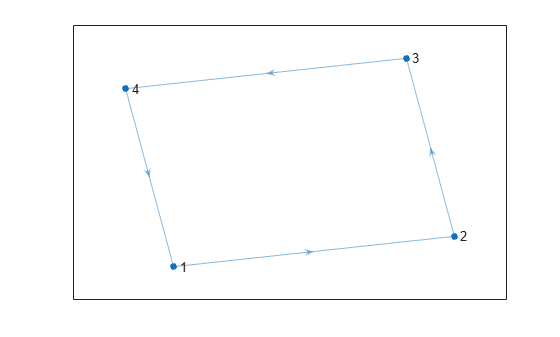Two graphs with the same reachability also have the same transitive reduction. Therefore, any cycle of four nodes produces the same transitive reduction as H.

Create a directed graph that contains a different four node cycle: (1,3,4,2,1).

G1 = digraph([1 3 4 2],[3 4 2 1]);
plot(G1)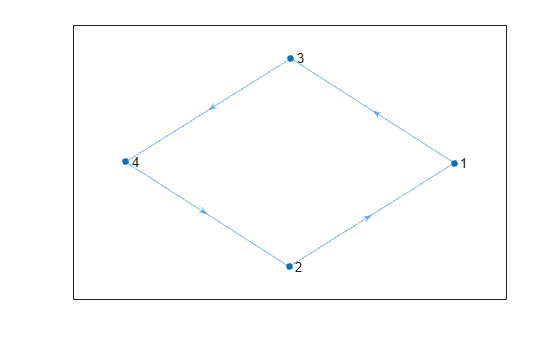Find the transitive reduction of G1. The cycle in G1 is reordered so that the transitive reductions H and H1 have the same cycle, (1,2,3,4,1).

H1 = transreduction(G1);
plot(H1)Create and plot a directed acyclic graph.

s = [1 1 1 1 2 3 3 4];
t = [2 3 4 5 4 4 5 5];
G = digraph(s,t);
plot(G)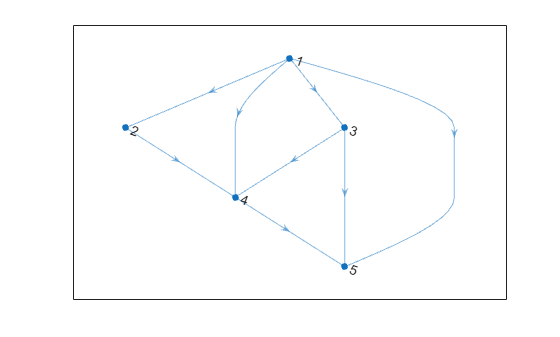Confirm that G does not contain any cycles.

tf = isdag(G)
tf = logical
1

Find the transitive reduction of the graph. Since the graph does not contain cycles, the transitive reduction is unique and is a subgraph of G.

H = transreduction(G);
plot(H)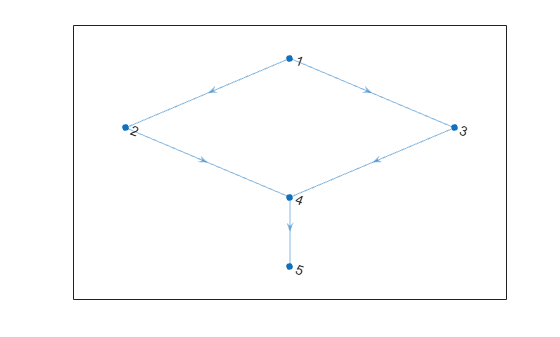Input Arguments

collapse all

Input graph, specified as a digraph object. Use digraph to create a directed graph object.

Example: G = digraph([1 2],[2 3])

Output Arguments

collapse all

Transitive reduction of G, returned as a digraph object. The table G.Nodes is copied to H, but any properties in G.Edges are dropped. H might contain new edges not present in G.

H contains the fewest number of edges that still preserve the reachability of graph G. In other words, transclosure(H) is the same as transclosure(G).

If isdag(G) is true, then H is unique and is a subgraph of G.